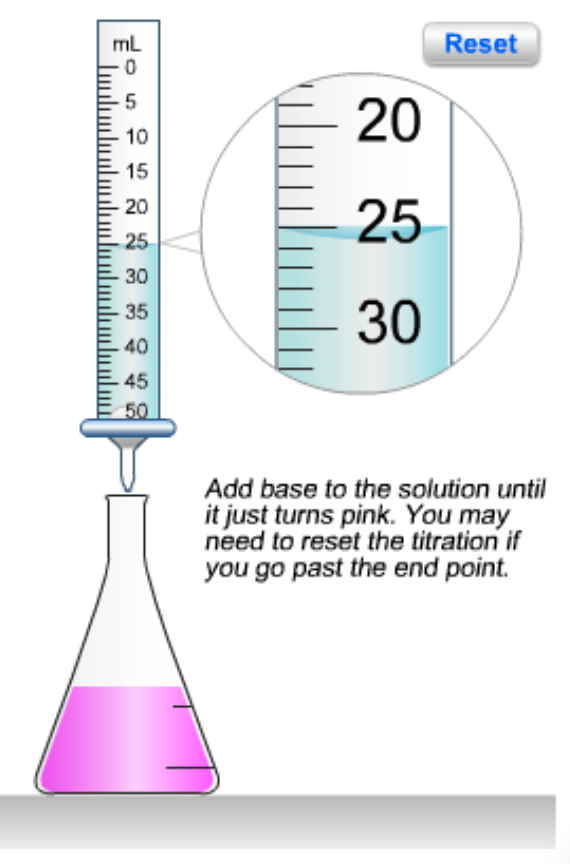# Problem: The flask shown here contains 0.365 g of acid and a few drops of phenolphthalein indicator dissolved in water. The buret contains 0.220 M NaOH. What volume (mL) of base is needed to reach the end point of the titration? What is the molar mass (g/mol) of the acid (assuming it is diprotic and that the end point corresponds to the second equivalence point)?

🤓 Based on our data, we think this question is relevant for Professor Owuor's class at ULL.

###### FREE Expert Solution

A diprotic acid reacting with NaOH will appear as:
H2A + 2NaOH --> 2H2O + Na2A
From the diagram, 25 mL of base (NaOH) has been added to reach the endpoint###### Problem Details

The flask shown here contains 0.365 g of acid and a few drops of phenolphthalein indicator dissolved in water. The buret contains 0.220 M NaOH.

What volume (mL) of base is needed to reach the end point of the titration?

What is the molar mass (g/mol) of the acid (assuming it is diprotic and that the end point corresponds to the second equivalence point)?What scientific concept do you need to know in order to solve this problem?

Our tutors have indicated that to solve this problem you will need to apply the Titrations of Diprotic and Polyprotic Acids concept. You can view video lessons to learn Titrations of Diprotic and Polyprotic Acids. Or if you need more Titrations of Diprotic and Polyprotic Acids practice, you can also practice Titrations of Diprotic and Polyprotic Acids practice problems.

What is the difficulty of this problem?

Our tutors rated the difficulty ofThe flask shown here contains 0.365 g of acid and a few drop...as medium difficulty.

How long does this problem take to solve?

Our expert Chemistry tutor, Dasha took 6 minutes and 39 seconds to solve this problem. You can follow their steps in the video explanation above.

What professor is this problem relevant for?

Based on our data, we think this problem is relevant for Professor Owuor's class at ULL.